# 【算法分析】QQ“一键退朝”之详细计算方法# 1.得到连心线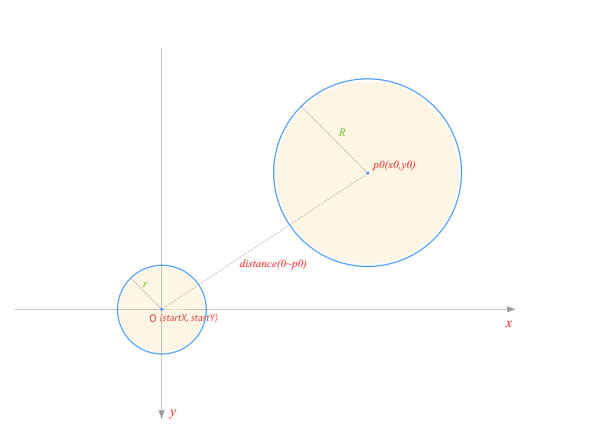PS：在移动端的坐标系 y$y$ 轴是向下的。

# 2.求切点坐标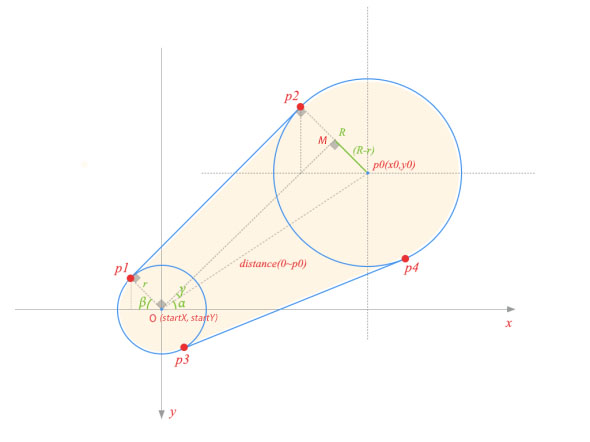sinγ=sinMOP0=MP0OP0=Rrd

γ=MOP0=arcsinRrd

tanα=startYy0x0startX

α=arctanstartYy0x0startX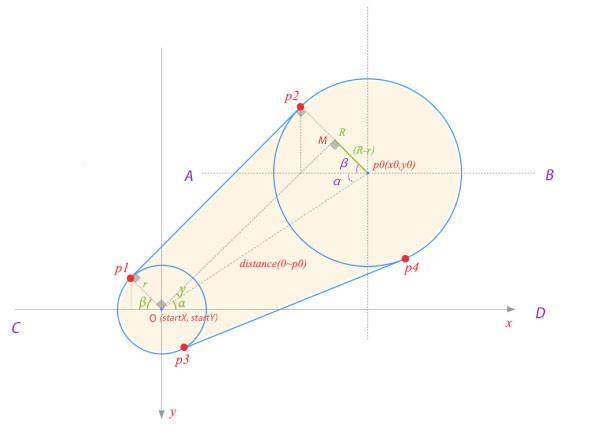AB//CDP0OD=α

AP0O=α

P1OC=βP1O//P2P0

P2P0A=P1OC=β

OMP2P0OMP0=90°π2

β=π2γα

P1.x=startXcosβrP1.y=startYsinβr

P2.x=x0cosβRP1.y=y0sinβR

# 3.求剩下两个切点的坐标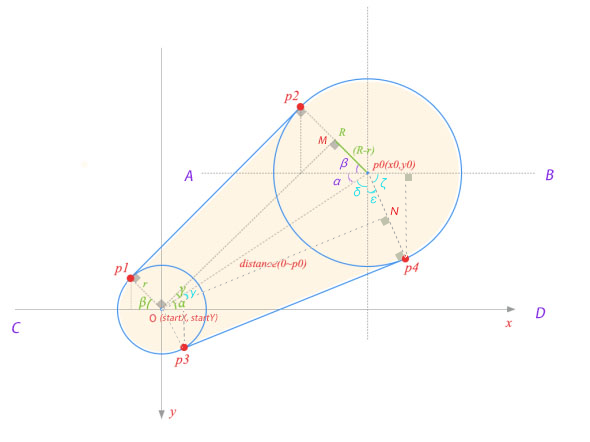α 阿尔法 β 贝塔 γ 伽玛 δ 德尔塔 ε 伊普西隆 ζ 泽塔

P0ON=γ

δ+α=90°π2

δ=π2α

ONP0P4

γ+δ+ε=π2ε=π2γδ

ε+ζ=π2

ζ=π2ε=π2π2γδ=π2π2γπ2α

ζ=π2+γα

P3.x=startX+cosζrP3.y=startY+sinζr

P4.x=x0+cosζRP4.y=y0+sinζR

# 4.画贝塞尔曲线

## 4.1 科普贝塞尔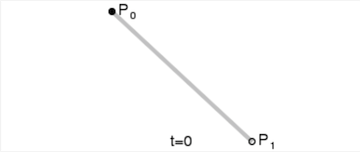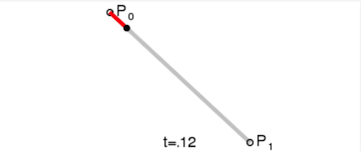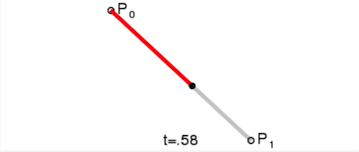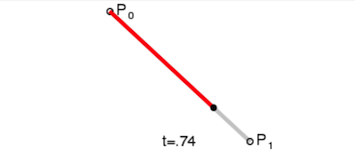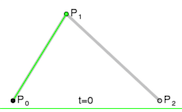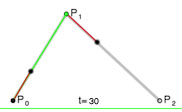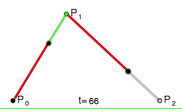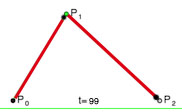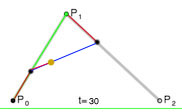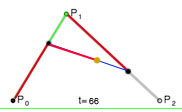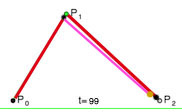## 4.2.寻找控制点References：

《QQ手机版 5.0“一键下班”设计小结》

《【Android开源项目解析】QQ“一键下班”功能实现解析——学习Path及贝塞尔曲线的基本使用》